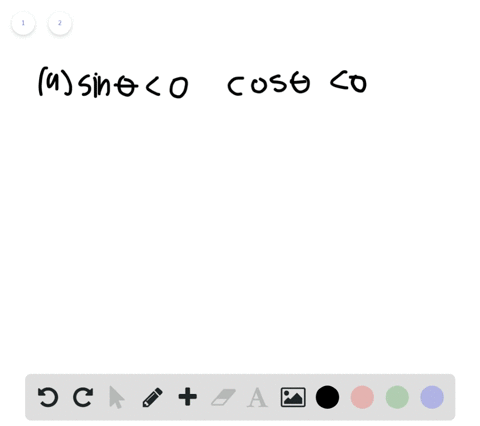🎉 The Study-to-Win Winning Ticket number has been announced! Go to your Tickets dashboard to see if you won! 🎉View Winning Ticket### Determining a Quadrant In Exercises 29 and 30 , d…

02:53University of Southern California
Problem 28

# Evaluating Trigonometric Functions Using TechnologyIn Exercises $25-28$ , use a calculator to evaluate each trigonometric function. Round your answers to four decimal places.$$\begin{array}{l}{\text { (a) } \cot (1.35)} \\ {\text { (b) } \tan (1.35)}\end{array}$$

## Discussion

You must be signed in to discuss.

## Video Transcript

this problem asks us to use our calculator to evaluate both of the following triggered a metric functions. And then after we've evaluated them, we're supposed to round our answer to four decimal places. Now, when we first look at these two problems, the first thing we need to notice is that our angle measures are not in degrees, but they're in radiance. So for the 1st 1 we have to calculate co tangent and co tangent is simply the reciprocal of tangent. So Cho tangent of 1.35 Sampley equals one over tangent of 1.35 radiance. And when you plug that into your calculator, you're going to find that that answer round into four decimal places. Zero 0.22 for fine. Now, to do part B, all you have to do is plug tangent of 1.35 into your calculator and you'll find that that answer right there is equal to 4.4 552 and there you have it. Those are both those angles evaluated to four decimal places